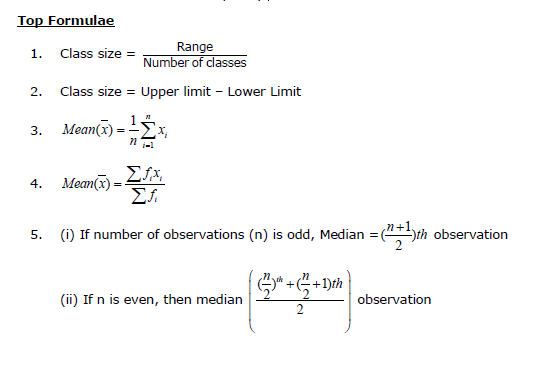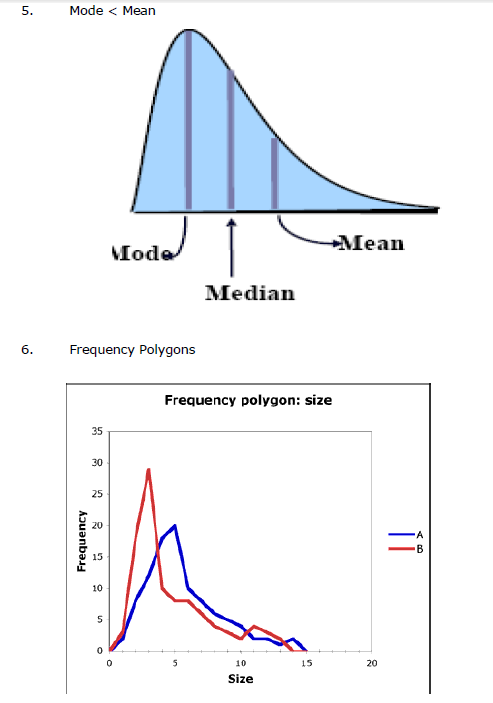# CBSE Class 9 Mathematics Statistics Notes

Download CBSE Class 9 Mathematics Statistics Notes in PDF format. All Revision notes for Class 9 Mathematics have been designed as per the latest syllabus and updated chapters given in your textbook for Mathematics in Standard 9. Our teachers have designed these concept notes for the benefit of Grade 9 students. You should use these chapter wise notes for revision on daily basis. These study notes can also be used for learning each chapter and its important and difficult topics or revision just before your exams to help you get better scores in upcoming examinations, You can also use Printable notes for Class 9 Mathematics for faster revision of difficult topics and get higher rank. After reading these notes also refer to MCQ questions for Class 9 Mathematics given our website

## Statistics Class 9 Mathematics Revision Notes

Class 9 Mathematics students should refer to the following concepts and notes for Statistics in standard 9. These exam notes for Grade 9 Mathematics will be very useful for upcoming class tests and examinations and help you to score good marks

### Statistics Notes Class 9 Mathematics

CBSE Class 9 Concepts for Statistics. Learning the important concepts is very important for every student to get better marks in examinations. The concepts should be clear which will help in faster learning. The attached concepts made as per NCERT and CBSE pattern will help the student to understand the chapter and score better marks in the examinations.

Chapter 14

Statistics

Chapter Notes

Top Definitions

1. Facts or figures collected with a definite purpose are called data.

2. Statistics deals with collection, presentation, analysis and interpretation of numerical data.

3. Arranging data in a order to study their salient features is called presentation of data.

4. Data arranged in ascending or descending order is called arrayed data or an array.

5. When an investigator with a definite plan or design in mind collects data first handedly, it is called primary data.

6. Data when collected by someone else, say an agency or an investigator, comes to you, is known as the secondary data.

7. Variable is a quantity that assumes different values.

8. Range of the data is the difference between the maximum and the minimum values of the observations.

9. The small groups obtained on dividing all the observations are called classes or class intervals and the size is called the class size or class width.

10. Class mark of a class is the mid value of the two limits of that class.

11. A bar graph is the diagram showing a system of connections or interrelations between two or more things by using bars.

12. A histogram is the bar graph such that the area over each class interval is proportional to the relative frequency of data within this interval.

13. The number of times an observation occurs in the data is called the frequency of the observation.

14. A frequency distribution in which the upper limit of one class differs from the lower limit of the succeeding class is called an Inclusive or discontinuous Frequency Distribution.

15. A frequency distribution in which the upper limit of one class coincides from the lower limit of the succeeding class is called an exclusive or continuous Frequency Distribution.

16. A bar graph is a pictorial representation of data in which rectangular bars of uniform width are drawn with equal spacing between them on one axis, usually the x axis. The value of the variable is shown on the other axis that is the y axis.

17. A histogram is a set of adjacent rectangles whose areas are proportional to the frequencies of a given continuous frequency distribution.

18. The Cumulative Frequency of a class-interval is the sum of frequencies of that class and the classes which precede (come before) it.

19. The mean value of a variable is defined as the sum of all the values of the variable divided by the number of values.

20. Median is the value of middle most observation(s).

21. Mode of a statistical data is the value of that variate which has the maximum frequency.

Top Concepts

1. In case of continuous frequency distribution, the upper limit of a class is not to be included in that class while in discontinuous both the limits are included.

2. The height of rectangles corresponds to the numerical value of the data.

3. Frequency polygons are a graphical device for understanding the shapes of distributions.

4. Bar charts are used for comparing two or more values.

5. A histogram differs from a bar chart, as in the former it is the area of the bar that denotes the value, not the height.

6. The height of the rectangle as the ratio of the frequency of the class to the width or size of the class.

7. Last cumulative frequency is always the sum total of all the frequencies.

8. If both a histogram and a frequency polygon are to be drawn on the same graph, then we should first draw the histogram and then join the mid-points of the tops of the adjacent rectangles in the histogram with line-segments to get the frequency polygon.

9. If classes are not of equal width, then the height of the rectangle is calculated by the ratio of the frequency of that class, to the width of that class.

10. A measure of central tendency tries to estimate the central value which represents the entire data.

11. The three measures of central tendency for ungrouped data are mean, mode and median.

12. The disadvantage of arithmetic mean is that it is affected by extreme values.

13. The median is to be calculated only after arranging the data in ascending order or descending order.

22. Average height is the modal value.

23. Disadvantage of the mode is that it is not uniquely defined in many cases.

24. The data is symmetric about the mean position when the three averages mean median and mode are all equal.

25. The data is asymmetric when the three measures are unequal.

14. The variate corresponding to the highest frequency is to be taken as the mode and not the frequency.## Tags:

Click for more Mathematics Study Material
 CBSE Class 9 Mathematics Number Systems Notes
 CBSE Class 9 Mathematics Coordinate Geometry Notes
 CBSE Class 9 Mathematics Linear Equations In Two Variables Notes Set A CBSE Class 9 Mathematics Linear Equations In Two Variables Notes Set B
 CBSE Class 9 Mathematics Lines And Angles Notes
 CBSE Class 9 Mathematics Triangles Notes
 CBSE Class 9 Mathematics Quadrilaterals Notes
 CBSE Class 9 Mathematics Area Of Parallelograms Notes
 CBSE Class 9 Mathematics Geometric Constructions Notes
 CBSE Class 9 Mathematics Herons Formula Notes
 CBSE Class 9 Mathematics Surface Areas And Volumes Notes
 CBSE Class 9 Mathematics Activities and Projects

## Latest NCERT & CBSE News

Read the latest news and announcements from NCERT and CBSE below. Important updates relating to your studies which will help you to keep yourself updated with latest happenings in school level education. Keep yourself updated with all latest news and also read articles from teachers which will help you to improve your studies, increase motivation level and promote faster learning

### CBSE Term 2 Board Examinations

CBSE vide Circular No.Acad-51/2021 dated 5th July, 2021, notified that in the session 2021-2022, Board Examinations would be conducted in two terms, i.e.. Term I and Term II. This decision was taken due to the uncertainty arising out of COVID 19 Pandemic. Term I...

### Class 10th and 12th Term 2 Revaluation Process 2022

Evaluation of the Answer Books is done under a well-settled Policy. To ensure that the evaluation is error free, CBSE is taking several steps. After strictly following these steps, the result is prepared. Though, CBSE is having a well-settled system of assessment,...

### Board Exams Date Sheet Class 10 and Class 12

Datesheet for CBSE Board Exams Class 10  (Scroll down for Class 12 Datesheet) Datesheet for CBSE Board Exams Class 12

### Celebration of Matribhasha Diwas Mother Language day

UNESCO has declared 21st February of every year to be celebrated as International Mother Language day to promote dissemination of Mother Language of all, create awareness of linguistic and cultural traditions and diversity across the world and to inspire solidarity...